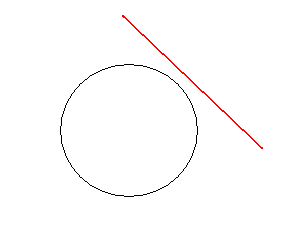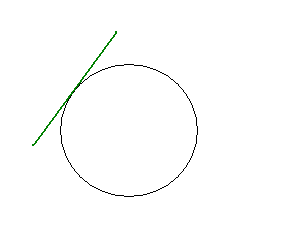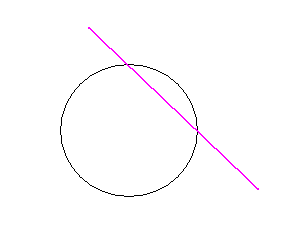# A line in the plane: positions regarding a circumference

A line on the plane, regarding a circumference, can be:

• Exterior to the circumference, if they have no point in common and the distance from the center to the line is greater than the length of the radius.• Tangent to the circumference, if the line touches at a point (the point of tangency) and the distance from the center to the line is equal to the length of the radius. A line tangent to a circle is perpendicular to the radius that connects the point of contact with the center.• Secant to the circumference, if they have two common points, that is, if the line cuts through the circumference at two different points and the distance from the center to the line is less than the length of the radius.